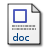# Development of a hybrid algorithm for efficiently solving mixed integer-continuous optimization problems

Liu, Dianzi (2016) Development of a hybrid algorithm for efficiently solving mixed integer-continuous optimization problems. Applied and Computational Mathematics, 5 (3). pp. 107-113. ISSN 2328-5605Microsoft Word (full paper_ by D LIU to PURE) - Accepted Version Download (160kB)

## Abstract

Problems with mixed integer-continuous design variables are a class of complicated optimization problems that commonly exist in practical engineering design work. In this paper, a hybrid algorithm combining metamodel-based Multipoint Approximation Method (MAM) and Hooke-Jeeves direct search technique is presented to efficiently seek the optimum solutions for mixed integer-continuous optimization problems. First, optimal continuous values are obtained by the Sequential Quadratic Programming method (SQP) on the approximated functions in a current trust region. Then, continuous values are rounded to the nearest integer values for discrete variables. Utilizing integer values as a starting point, the Hooke-Jeeves assisted MAM is applied to search for the discrete optimal solution in the sub-space of discrete variables as well as accordingly update the sub-optimal values for continuous design variables by SQP. The proposed hybrid algorithm is examined by the well established benchmark example and the obtained results demonstrate the superiority of the developed algorithm over GA in terms of computational cost and the quality of solutions.

Item Type: Article integer-continuous optimization,multipoint approximation method,metamodel,direct search,hybrid algorithm Faculty of Science > School of Mathematics Pure Connector 14 Oct 2016 09:00 18 Oct 2022 14:31 https://ueaeprints.uea.ac.uk/id/eprint/60928 10.11648/j.acm.20160503.13View Item